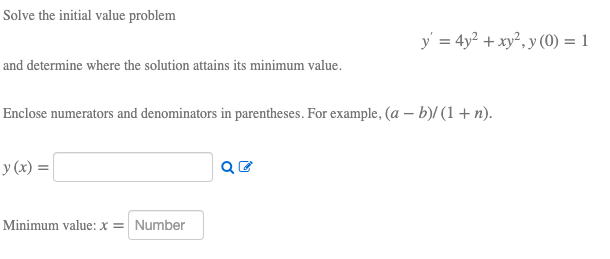# Question Solved1 AnswerSolve the initial value problem y' = 4y2 + xy2, y(0) = 1 and determine where the solution attains its minimum value. Enclose numerators and denominators in parentheses. For example, (a - b)/(1+n). y(x) = Qe Minimum value: x = NumberMOTBFI The Asker · Other MathematicsTranscribed Image Text: Solve the initial value problem y' = 4y2 + xy2, y(0) = 1 and determine where the solution attains its minimum value. Enclose numerators and denominators in parentheses. For example, (a - b)/(1+n). y(x) = Qe Minimum value: x = Number
More
Transcribed Image Text: Solve the initial value problem y' = 4y2 + xy2, y(0) = 1 and determine where the solution attains its minimum value. Enclose numerators and denominators in parentheses. For example, (a - b)/(1+n). y(x) = Qe Minimum value: x = Number